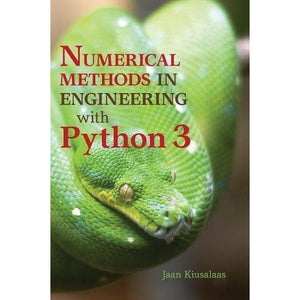># Numerical Methods in Engineering with Python 3

• £37.39
• Save £43

Jaan Kiusalaas
Cambridge University Press
Edition: 3, 2013-01-21
EAN 9781107033856, ISBN10: 1107033853

Paperback, 432 pages, 25.7 x 18.5 x 1.8 cm

This book is an introduction to numerical methods for students in engineering. It covers solution of equations, interpolation and data fitting, solution of differential equations, eigenvalue problems and optimisation. The algorithms are implemented in Python 3, a high-level programming language that rivals MATLAB® in readability and ease of use. All methods include programs showing how the computer code is utilised in the solution of problems. The book is based on Numerical Methods in Engineering with Python, which used Python 2. This new edition demonstrates the use of Python 3 and includes an introduction to the Python plotting package Matplotlib. This comprehensive book is enhanced by the addition of numerous examples and problems throughout.